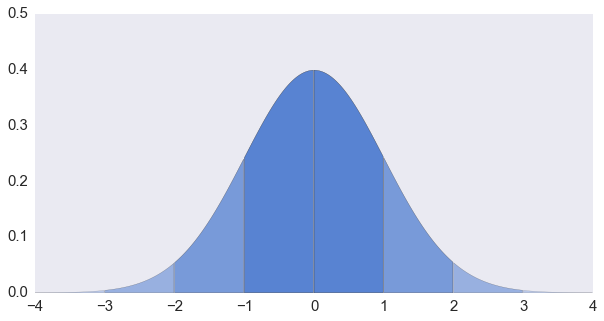# 正态分布随机数的生成 (2)

## 接受—拒绝法# VMware Player 升级 / 卸载报错

The MSI '' failed

# 正态分布随机数的生成 (1)

$$f(x \; | \; \mu, \sigma) = \frac{1}{\sigma\sqrt{2\pi} } \; e^{ -\frac{(x-\mu)^2}{2\sigma^2} }$$Continue reading “正态分布随机数的生成 (1)”